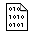## Firefly and PC GAMESS-related discussion club

Learn how to ask questions correctlyRe^5: Can not optimize geometry in MP2/aug-cc-pVDZ

Alexander Vasyanin
avasyanin@gmail.com

Dear Thomas!

I've tried to disable DLC (see attached output), but also without success:

Here are 20 first iterations:
grep RMS Karma56OH-mp2-d5-opt.dat |cat -n |head -20
1  E=     -727.7564447892  GMAX=   0.0017745  GRMS=   0.0003867
2  E=     -727.7564469648  GMAX=   0.0033305  GRMS=   0.0007018
3  E=     -727.7564682074  GMAX=   0.0009339  GRMS=   0.0002587
4  E=     -727.7564710925  GMAX=   0.0013823  GRMS=   0.0003371
5  E=     -727.7564797897  GMAX=   0.0006508  GRMS=   0.0001646
6  E=     -727.7564826106  GMAX=   0.0009965  GRMS=   0.0002087
7  E=     -727.7564867291  GMAX=   0.0003811  GRMS=   0.0001103
8  E=     -727.7564878238  GMAX=   0.0007359  GRMS=   0.0001514
9  E=     -727.7564885245  GMAX=   0.0002804  GRMS=   0.0000891
10  E=     -727.7564891625  GMAX=   0.0004215  GRMS=   0.0000810
11  E=     -727.7564896416  GMAX=   0.0004183  GRMS=   0.0000890
12  E=     -727.7564900567  GMAX=   0.0003822  GRMS=   0.0000824
13  E=     -727.7564906809  GMAX=   0.0003616  GRMS=   0.0000840
14  E=     -727.7564913691  GMAX=   0.0003790  GRMS=   0.0000919
15  E=     -727.7564919013  GMAX=   0.0005374  GRMS=   0.0000995
16  E=     -727.7564921004  GMAX=   0.0001675  GRMS=   0.0000519
17  E=     -727.7564922661  GMAX=   0.0004710  GRMS=   0.0000773
18  E=     -727.7564909438  GMAX=   0.0006154  GRMS=   0.0001530
19  E=     -727.7564924073  GMAX=   0.0005384  GRMS=   0.0000735
20  E=     -727.7564924563  GMAX=   0.0004197  GRMS=   0.0000707

and 20 last:

grep RMS Karma56OH-mp2-d5-opt.dat |cat -n |tail -20
167  E=     -727.7564939108  GMAX=   0.0004250  GRMS=   0.0000655
168  E=     -727.7564939269  GMAX=   0.0002935  GRMS=   0.0000612
169  E=     -727.7564937578  GMAX=   0.0003022  GRMS=   0.0000709
170  E=     -727.7564939269  GMAX=   0.0004125  GRMS=   0.0000621
171  E=     -727.7564939187  GMAX=   0.0005626  GRMS=   0.0000976
172  E=     -727.7564939448  GMAX=   0.0002341  GRMS=   0.0000442
173  E=     -727.7564939459  GMAX=   0.0003595  GRMS=   0.0000584
174  E=     -727.7564938032  GMAX=   0.0005515  GRMS=   0.0000849
175  E=     -727.7564939271  GMAX=   0.0003477  GRMS=   0.0000597
176  E=     -727.7564939483  GMAX=   0.0001578  GRMS=   0.0000333
177  E=     -727.7564939576  GMAX=   0.0004467  GRMS=   0.0000706
178  E=     -727.7564938031  GMAX=   0.0006593  GRMS=   0.0000969
179  E=     -727.7564938967  GMAX=   0.0004309  GRMS=   0.0000837
180  E=     -727.7564934756  GMAX=   0.0004685  GRMS=   0.0000923
181  E=     -727.7564939480  GMAX=   0.0003942  GRMS=   0.0000727
182  E=     -727.7564939279  GMAX=   0.0002317  GRMS=   0.0000473
183  E=     -727.7564938419  GMAX=   0.0003029  GRMS=   0.0000609
184  E=     -727.7564937971  GMAX=   0.0003647  GRMS=   0.0000763
185  E=     -727.7564939054  GMAX=   0.0003855  GRMS=   0.0000595
186  E=     -727.7564938933  GMAX=   0.0003679  GRMS=   0.0000795

All used options can be found at the head of file.

Thanks,
Alexander

On Wed Feb 25 '15 7:22pm, Thomas Pijper wrote
---------------------------------------------
>Dear Alex and Alexander,

>I've already started a few tests. One them has DLCs disabled and seems to converge (it's still running) without using outrageously tight convergence criteria. I currently speculate that something is going wrong with the generation of the DLCs, either because of the use of IFDMOD=2 (which is of course experimental) and/or the presence of two intramolecular hydrogen bonds.
>
>
>Kind regards,
>Thom
>
>
>
>On Wed Feb 25 '15 6:52pm, Alex Granovsky wrote
>----------------------------------------------
>>Dear Alexander,
>>Dear Thom,

>>I'm sorry for delay with my feedback. I was waiting for CPU resources to play with this job.
>>I'm now investigate convergence problem and will report my findings in a couple of days.

>>Kind regards,
>>Alex
>>
>>
>>
>>On Wed Feb 25 '15 11:15am, Thomas Pijper wrote
>>----------------------------------------------
>>>Dear Alexander,

>>>Thanks for the followup. Sorry to hear that further tightening of accuracy options did not help. I'm actually surprised this system gives any problems as it is seemingly quite straightforward (a stable neutral organic molecule with a geometry close to equilibrium).

>>>If you like, I can experiment a bit with your system though this will take some time.
>>>
>>>
>>>Kind regards,
>>>Thom
>>>
>>>
>>>
>>>On Tue Feb 24 '15 9:22pm, Alexander Vasyanin wrote
>>>--------------------------------------------------
>>>>Hi All!

>>>>I didn't solve the problem. Looks like it appears when diffusion functions added to the basis sets: with 6-311G* and cc-pVDZ it optimizes, but 6-311+G* and aug-cc-pVDZ both failed, oscillating with GMAX not less than 1E-4.
>>>>
>>>>
>>>>I think the problem is with some gradient artifacts so I also tried to set tighter values to NCONV (8) in addition to values for \$MP2GRD recommended by Prof. Granovsky, but it breaks SCF convergence.

>>>>So I gave up...
>>>>
>>>>
>>>>On Mon Feb 9 '15 7:47pm, Alexander Vasyanin wrote
>>>>-------------------------------------------------
>>>>>Dear All,
>>>>>I've got a problem with geometry optimization (see attached input file). DFT methods (B3LYP, PBE0) with the same input geometry converge fine, but MP2 fails to converge showing a progress like this:

>>>>>\$ grep 'E=' 1.dat | cat -n
>>>>>     1  E=     -727.7926962177  GMAX=   0.0001417  GRMS=   0.0000243

>>>>>I've tried to use symmetry (CS) to make calculation faster with IHREP=5 option, but it also didn't help to reach convergence even after 40 iterations.

>>>>>Is it possible to improve convergence (may be with some option in \$MP2GRD)?This message contains the 3846 kb attachment [ output_3.zip ]Wed Feb 25 '15 7:46pmThis message read 532 times